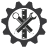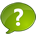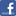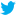SuperTeacherTools### Speed Match Review Game

This game has been played 28 times
The best time ever was Jesse G at 83 seconds.
The best time today is at seconds.
How To Play:
Drag the squares in the gray area and drop them in the matching squares in the blue area below.
If you drop a square in the correct spot, it will disappear.
If you make a mistake, the square will return to its original spot.
Good luck!

Let's Play Speed Match!

12
15

<

4

-5.7, -5 3/5, 5.43, 5 5/11

>

x-axis: (-1,-5) y-axis: (1,5)

-19 feet

-0.68
(3,-6)

<

see graph; 8 units
96

-20

2

-0.63636363....

-9.40, -9 3/11, -9 1/5, 9.22

>

-12, -9, -3, 0, 4
5, 2, -6, -8, -15
11

(-4,-5)

see number line
What is the reflection point of point B (-3,-6) across the y-axis?

Write an integer to describe the situation:

The balloon expands two centimeters each second.

Write an integer to describe the situation:

Stacey lost a twenty dollar bill.

Write -7/11 as a decimal.

Replace each blank with <, >, or = to make a true sentence:

– 7 _____ -6

Replace each blank with <, >, or = to make a true sentence:

| –6| ____ |9|

Order –9, 4 –12, 0, and -3 from least to greatest.
144 in = _______ ft (12 in = 1 ft)
Write -17/25 as a decimal.

Order the numbers below from least to greatest:

5 5/11, -5.7, 5.43, -5 3/5

The locations of three fish relative to the water’s surface are
–25 feet, –31 feet, and -19 feet. Which distance has the least absolute value?

In which quadrant is the point at (–6, -2) located?

Evaluate: | –9| – |–5|

Order the numbers below from least to greatest:

-9 1/5, 9.22, -9 3/11,-9.40

Reflect point C (-1,5) across the x and y axes. Write down the new reflection points.
Evaluate: | –8| + |–7|

What is the reflection point of point A (-4,5) across the x-axis?

In which quadrant is the point at (–4, 2) located?
Order –6, 5 –15, 2, and -8 from greatest to least.
6 lbs = ______ oz (16 oz = 1 lb)

Replace each blank with <, >, or = to make a true sentence:

| –5| ______– 5

Graph and label the points A (5, -3), B (–3, -3), and C (–2, –4) on a coordinate plane. Then find the distance between points A and B. The distance between points A and B is ______units.

Replace each blank with <, >, or = to make a true sentence:

–4 ____–15

88 fl oz = _____ c (1 c = 8 fl oz)
Graph the set {–3, –4, -2, 3} on a number line.